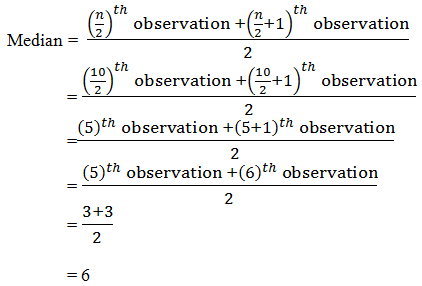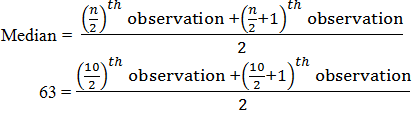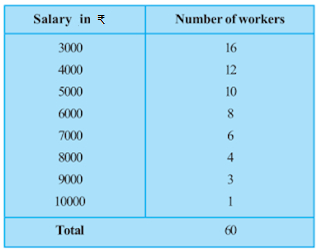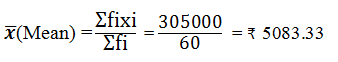### NCERT Solutions For Class 9 Chapter – 14 Exercise – 14.4

1. The following number of goals were scored by a team in a series of 10 matches:

2, 3, 4, 5, 0, 1, 3, 3, 4, 3

Find the mean, median and mode of these scores.

Solution:

Mean = Average = Sum of all the observations/Total number of observations

= (2+3+4+5+0+1+3+3+4+3)/10

= 28/10

= 2.8

Median,

To find the median, we first arrange the data in ascending order.

0, 1, 2, 3, 3, 3, 3, 4, 4, 5

Here,

Number of observations (n) = 10

Since the number of observations are even, the median can be calculated as:= 3

Mode,

To find the mode, we first arrange the data in ascending order.

0, 1, 2, 3, 3, 3, 3, 4, 4, 5.

Here,

We find that 3 occurs most frequently (4 times)

∴ Mode = 3

2. In a mathematics test given to 15 students, the following marks (out of 100) are recorded:

41, 39, 48, 52, 46, 62, 54, 40, 96, 52, 98, 40, 42, 52, 60
Find the mean, median and mode of this data.

Solution:

Mean=Average = Sum of all the observations/Total number of observations

= (41+39+48+52+46+62+54+40+96+52+98+40+42+52+60)/15

= 822/15

= 54.8

Median,

To find the median, we first arrange the data in ascending order.

39, 40, 40, 41, 42, 46, 48, 52, 52, 52, 54, 60, 62, 96, 98

Here,

Number of observations (n) = 15

Since the number of observations are odd, the median can be calculated as:

Median = [(n+1)/2]th observation

= [(15+1)/2]th observation

= (16/2)th observation

= 8th observation

= 52

Mode,

To find the mode, we first arrange the data in ascending order.

39, 40, 40, 41, 42, 46, 48, 52, 52, 52, 54, 60, 62, 96, 98

Here,

We find that 52 occurs most frequently (3 times)

∴ Mode = 52

3. The following observations have been arranged in ascending order. If the median of the data is 63, find the value of x.

29, 32, 48, 50, x, x+2, 72, 78, 84, 95

Solution:

Number of observations (n) = 10

Given that Median = 63

Since the number of observations are even, the median can be calculated as:63 = [5th observation+(5+1)th observation]/2

63 = [5th observation+6th observation]/2

63 = (x+x+2)/2

63 = (2x+2)/2

x = 63-1

x = 62

4. Find the mode of 14, 25, 14, 28, 18, 17, 18, 14, 23, 22, 14, 18.

Solution:

Mode,

To find the mode, we first arrange the given data in ascending order.

14,14,14,14,17,18,18,18,22,23,25,28

Here,

We find that 14 occurs most frequently (4 times)

∴ Mode = 14

5. Find the mean salary of 60 workers of a factory from the following table:Solution:

 Salary (xi) Number of workers (fi) fixi 3000 16 48000 4000 12 48000 5000 10 50000 6000 8 48000 7000 6 42000 8000 4 32000 9000 3 27000 10000 1 10000 Total Σfi = 60 Σfixi = 305000, the mean salary is ₹5083.33

6. Give one example of a situation in which

(i) the mean is an appropriate measure of central tendency.

(ii) the mean is not an appropriate measure of central tendency but the median is an appropriate measure of central tendency.

Solution:

(i) Mean marks obtained in the examination

(ii) Runs scored by Mahendra Singh Dhoni in 7 matches are,

39, 51, 56, 102, 83, 48, 91

Here,

Mean = (39+51+56+102+83+48+91)/7

= 470/7

= 67.1.

Median,

Arranging in ascending order we get 39, 48 51, 56, 83, 91, 102

n = 7

Median = [(n+1)/2]th observation

= ( (7+1)/2)th observation

= (8/2)th observation

= 4th observation

= 56